# Adding And Subtracting Decimals - Definition with Examples

The Complete K-5 Math Learning Program Built for Your Child

• 40 Million Kids

Loved by kids and parent worldwide

• 50,000 Schools

Trusted by teachers across schools

• Comprehensive Curriculum

Aligned to Common Core

Decimal number

• A decimal number is a fraction whose denominator is a power of 10. For example, the fraction 410  can be written in decimal form as 0.4, where:

• The dot is called the decimal point.

• The number to the right of the decimal point denotes the numerator of the fraction, that is, the fractional part.

• The number to the left of the decimal point denotes the whole number part of the fraction.

• For example, 4 310  is expressed as 4.3, where 4 is the whole number part and 0.3 is the fractional part.

• The decimal point separates the whole number part from the fractional part.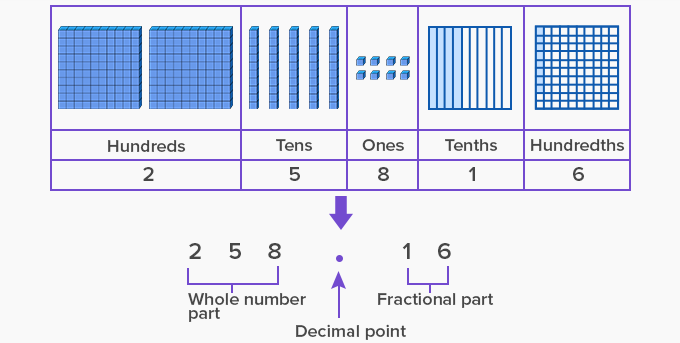Decimals in real life

We deal with decimal addition and subtraction in everyday life while dealing with:

• Money

• Measurements (Length, Mass, Capacity)

• Temperature

Decimals can be added or subtracted in the same way as we add or subtract whole numbers.

## Steps to add or subtract Decimals:

• Convert decimals to like decimals. (Decimals that have the same number of digits after the decimal point are like decimals).

• Write the decimals one below the other as per the places of the digits.

• Add or subtract starting from the rightmost digit and moving towards the leftmost digit.

• Place the decimal point under the decimal point in the answer.

Example: Add 23.45; 13.101 and 345.5

1.  Convert to like decimals: The highest decimal place is 3, so we add zeros in other numbers and get 3 decimal places in them too.

13.101

23.45 = 23.450

345.5 = 345.500

2.  Line up the decimals: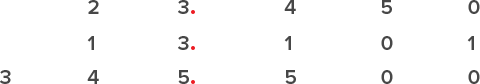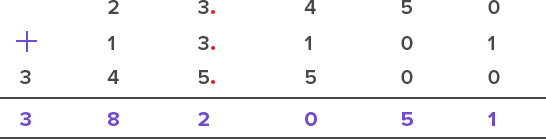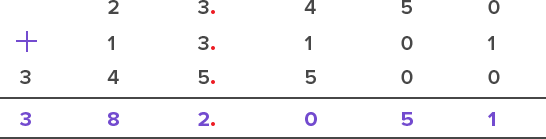Example: Kylie had 25 m of ribbon. She uses 8 m and 13 cm to decorate a skirt. How much ribbon is remaining with Kylie?

Solution:

Length of ribbon Kylie had = 25 m = 25.00

Length of ribbon used by Kylie = 8 m 13 cm = 8.13

The remaining length of ribbon = 25.00 – 8.13

= 16.87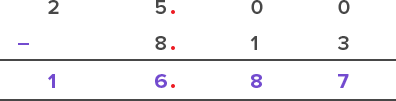Fun Facts The word “decimal” originated in the early 17th century from the Latin word “decimus” which means “tenth”. We use decimals when we want precise and accurate answers.

Won Numerous Awards & Honors